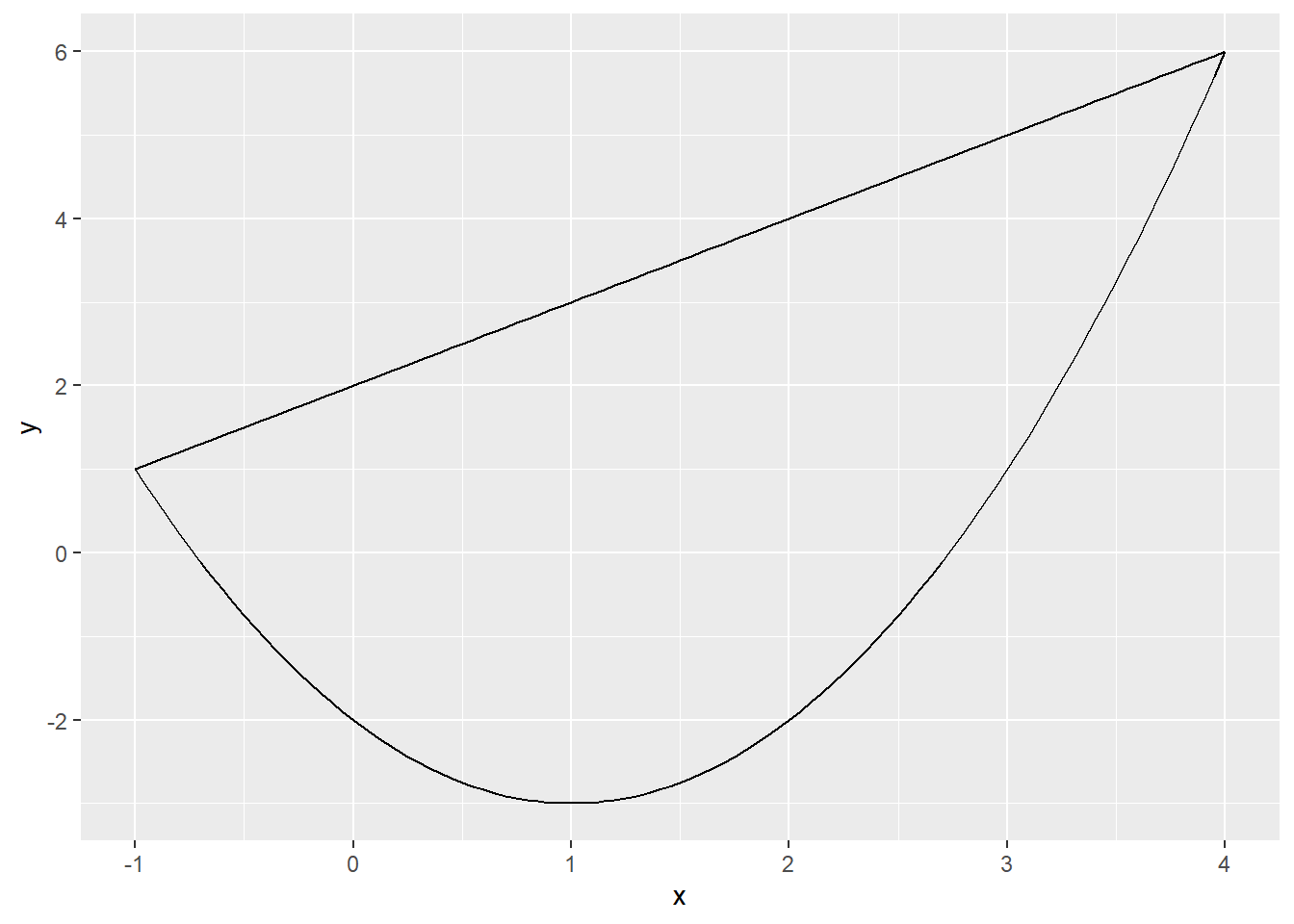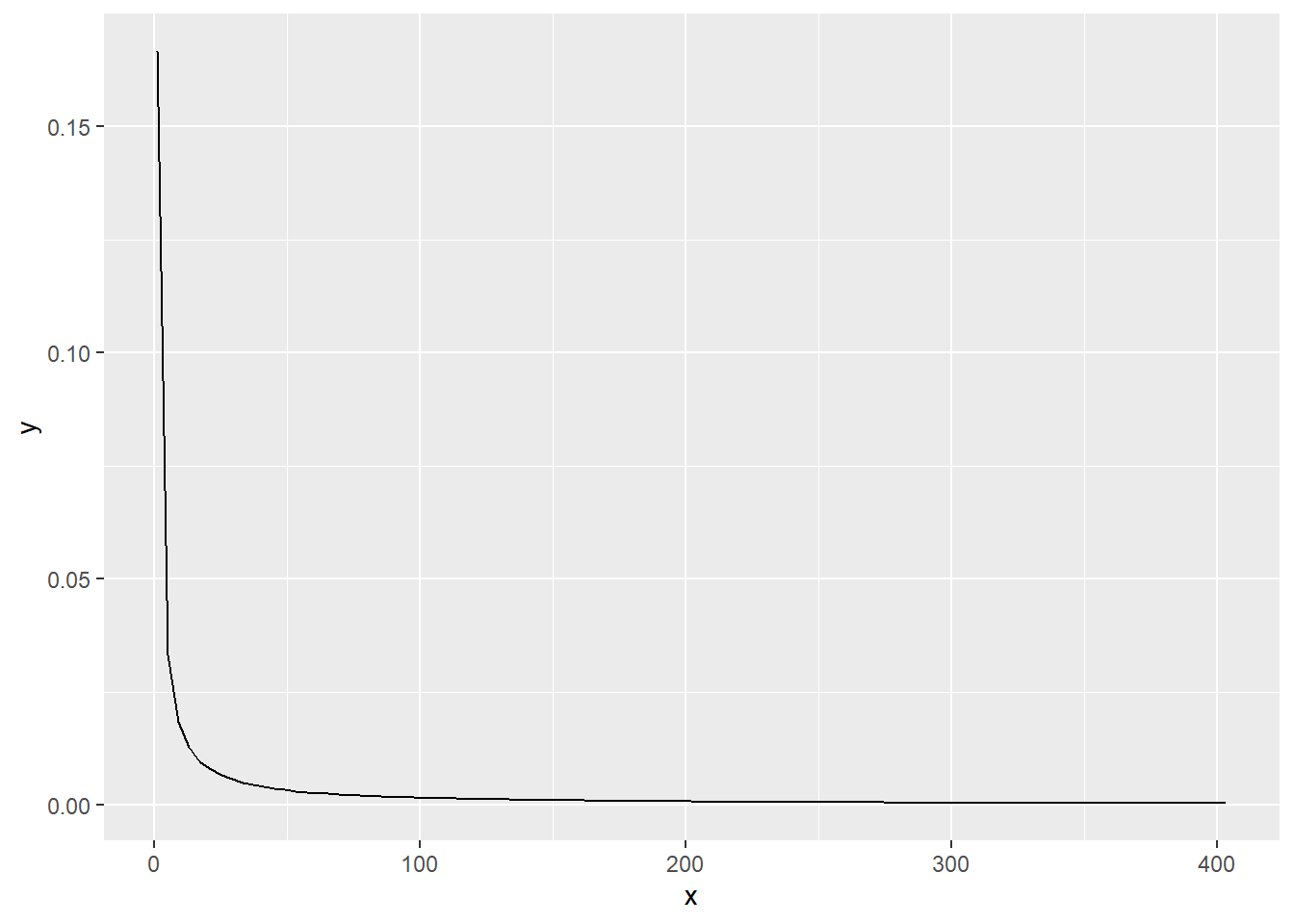# Calculus Assignment 13

## Question 1

Use integration by substition to solve the integral below.

$\int 4e^{-7x}dx$

Set the value to substitute: u = -7x

Find the value of dx: dx = -du/7

$\frac{-4}{7}\int e^{u}du$ $\frac{-4}{7}e^{u} + c$

## Question 2

Biologists are treating a pond contaminated with bacteria. The level of contamination is changing at a rate of dN/dt =  3150/t^4  220 bacteria per cubic centimeter per day, where t is the number of days since treatment began. Find a function N(t) to estimate the level of contamination if the level after 1 day was 6530 bacteria per cubic centimeter.

$\frac{dN}{dt} = \frac{-3150}{t^4} - 220$ $N(t) = \frac{1050}{t^3} - 220t + c$ $6530 = 1050 - 220 + c$ $c = 5700$

$N(t) = \frac{1050}{t^3} - 220t + 5700$

## Question 3

Find the total area of the red rectangles in the figure below, where the equation of the line is f(x) = 2x  9.

Midpoint rule: $M_n = \sum{f(m_i)\Delta x}$

f <- function(x) {
return((2*x)-9)
}
delta_x = 1
area = delta_x*(f(5) + f(6) + f(7) + f(8))
print("Area of rectangles")
##  "Area of rectangles"
print(area)
##  16

## Question 4

Find the area of the region bounded by the graphs of the given equations.

y = x^2  2x  2, y = x + 2 Enter your answer below.

$\int_{-1}^{4} (x+2) - (x^2  2x  2) dx$

$\int_{-1}^{4} x^2 + 3x + 4 dx$

$[\frac{x^3}{3} + \frac{3x^2}{2} + 4x]_{-1}^{4}$

y_1 <- function(x) {
return((x^2)-2*x-2)
}

y_2 <- function(x) {
return(x+2)
}

ggplot(data = data.frame(x = 0), mapping = aes(x = x)) +
stat_function(fun = y_1) +
stat_function(fun = y_2) + xlim(-1,4)y_int <- function(x) {
return(((x^3)/3) + 3*(x^2)/2 + 4*x)
}

print("Area of the region bounded by the graphs:")
##  "Area of the region bounded by the graphs:"
print(y_int(4)-y_int(-1))
##  64.16667

## Question 5

A beauty supply store expects to sell 110 flat irons during the next year. It costs $3.75 to store one flat iron for one year. There is a fixed cost of$8.25 for each order. Find the lot size and the number of orders per year that will minimize inventory costs.

Storage cost: $sc = 3.75 * \frac{x}{2}$

Ordering cost: $oc = 8.25 * \frac{110}{x}$

Total cost: $tc = 1.875x + \frac{907.5}{x}$

Derivative: $\frac{dtc}{dx} = 1.875- \frac{907.5}{x^2}$ $\frac{907.5}{1.875} = x^2$

$x = 22$

110 / 22 = 5 orders

## Question 6

Use integration by parts to solve the integral below. $\int ln(9x) * x^6 dx$

$u = ln(9x) | dv = x^6dx$

$du = \frac{1}{x} dx | v = \frac{x^7}{7}$

$\int u dv = uv - \int vdu$

$\int ln(9x) x^6dx = ln(9x) * \frac{x^7}{7} - \int \frac{x^7}{7} \frac{1}{x}dx$

$\int ln(9x) * x^6 dx = \frac{x^7ln(9x)}{7} - \frac{1}{7}\int x^6 dx$

$\int ln(9x) * x^6 dx = \frac{x^7ln(9x)}{7} - \frac{x^7}{49} + c$ $\int ln(9x) * x^6 dx = \frac{x^7(7ln(9x) - 1)}{49} + c$

## Question 7

Determine whether f(x) is a probability density function on the interval [1, e^6]. If not, determine the value of the definite integral.

f(x) = 1/6x

$\int_{1}^{e^6} {\frac{1}{6x}}$ $\frac{1}{6}[ln(x)]_{1}^{e^6}$

$\frac{1}{6}[ln(e^6)-ln(1)]$

$\frac{1}{6}[6 - 0] = 1$

f(x) is the probability density function on this interval since it is equal to 1.

pdf <- function(x) {
return(1/(6*x))
}

ggplot(data = data.frame(x = 0), mapping = aes(x = x)) +
stat_function(fun = pdf) + xlim(1, exp(6))